Courses

# Matrix MCQ - 3

## 30 Questions MCQ Test Topic-wise Tests & Solved Examples for IIT JAM Mathematics | Matrix MCQ - 3

Description
This mock test of Matrix MCQ - 3 for Mathematics helps you for every Mathematics entrance exam. This contains 30 Multiple Choice Questions for Mathematics Matrix MCQ - 3 (mcq) to study with solutions a complete question bank. The solved questions answers in this Matrix MCQ - 3 quiz give you a good mix of easy questions and tough questions. Mathematics students definitely take this Matrix MCQ - 3 exercise for a better result in the exam. You can find other Matrix MCQ - 3 extra questions, long questions & short questions for Mathematics on EduRev as well by searching above.
QUESTION: 1

Solution:
QUESTION: 2

Solution:
QUESTION: 3

### A matrix A such that A2 = I, is called

Solution:
QUESTION: 4

A matrix A such that Am = 0 for some positive integer and Ak ≠ 0 for any k < m, is said to be

Solution:
QUESTION: 5

A square matrix A such the AT = —A, is called a

Solution:

Transpose of a matrix happens to be equal to the negative of itself, then one can say that the matrix is skew symmetric.

QUESTION: 6

A square matrix A such that AT = -A, is called a

Solution:

In mathematics, particularly in linear algebra, a skew-symmetric matrix is a square matrix whose transpose equals its negative.

QUESTION: 7

Mark the in correct statement. If A* and B* are the transpose of the conjugates of A and B respectively, the n

Solution:
QUESTION: 8

A square matrix A is said to be Hermitian Matrix if

Solution:

matrix A = [aij] ∈ Mn is said to be Hermitian if A = A or
= aij, for aji only.

QUESTION: 9

A square matrix A is said to be Skew Hermitian Matrix, if

Solution:

matrix A = [aij] ∈ Mn is skew-Hermitian if A = − A *

QUESTION: 10

If A and B are two odd order Skew — symmetric matrices such that AB = BA, then what is the matrix AB?

Solution:
QUESTION: 11

Mark the incorrect statement

Solution:
QUESTION: 12

The diagonal elements of a Skew Hermitian Matrix are

Solution:
QUESTION: 13

To convert a Hermitian Matrix into Skew Hermitian Matrix, the Hermitian Matrix must be multiplied by

Solution:
QUESTION: 14

Which is not correct? If A is any square matrix, then... is Hermitian Matrix

Solution:
QUESTION: 15

Every square matrix is uniquely expressible as

Solution:
QUESTION: 16

If A is any square matrix, then A — A' is a

Solution:

(A − A')' = A' − (A')'

= A' − A

= −(A − A')
Therefore, it is a skew symmetric matrix

QUESTION: 17

If A and B are symmetric matrices of the same order, then which one of the following is not correct?

Solution:
QUESTION: 18

A square matrix A is said to be ... if AAT = I

Solution:
QUESTION: 19

If A =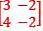satisfies the matrix equation A2 — kA + 21 = 0, then what is the value of k?

Solution:
QUESTION: 20

A square matirx A is said to be .... if A* A = I

Solution:
QUESTION: 21

Under which one of the following condition does the system of equations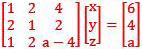have a unique solution?

Solution:
QUESTION: 22

One of the integrating factor of the differential equation
(y2 – 3xy)dx + (x2 – xy)dy = 0 is

Solution:

(y2 – 3xy)dx + (x2 – xy) dy = 0;
M = y2 – 3xy, N = x2 – xy
Here differential equation is homogeneous, then
Mx + Ny = xy2 – 3x2y + x2y – xy2 = – 2x2y ≠ 0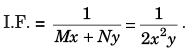QUESTION: 23

The product of two orthogonal matrices is a ... matrix

Solution:
QUESTION: 24

The product of two Unitary matrices is a ________ matrix

Solution:

the product of two unitary matrices is always unitary.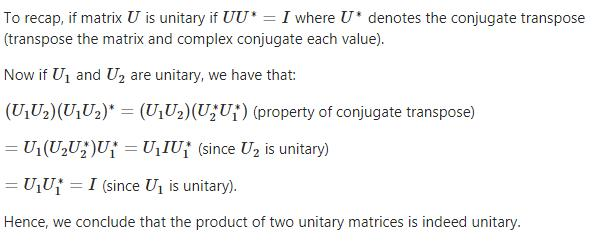QUESTION: 25

The points ( x1, y1 ) , ( x2, y2), ( x3, y3) are collinear if the rank of the matrix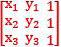is

Solution:
QUESTION: 26

A neccessary condition for the linear equations a1x + b1 = 0 and a2x + b2 = 0, to have a common solution is that

Solution:
QUESTION: 27

A necesary and sufficient condition for the linear equations a1x + b1y + c1 = 0 and a2x + b2y + c2 = 0 to possess a unique solution is that

Solution:
QUESTION: 28

The value of the determinant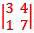is

Solution:
QUESTION: 29

Which of the following statement is incorrect?

Solution:
QUESTION: 30

Let A and B be any two n x n matrices and tr(A) =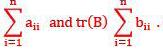Consider the following statement
I. tr(AB) = tr(BA)
II. tr(A + B) = tr. (A) + tr(B)
Which of the following statement given above is/are correct?

Solution: